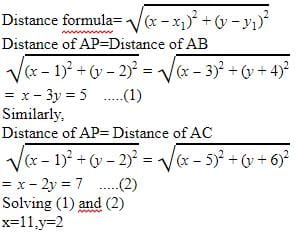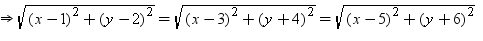# Test: Coordinate Geometry - 1

## 25 Questions MCQ Test Mathematics (Maths) Class 9 | Test: Coordinate Geometry - 1

Description
Attempt Test: Coordinate Geometry - 1 | 25 questions in 25 minutes | Mock test for Class 9 preparation | Free important questions MCQ to study Mathematics (Maths) Class 9 for Class 9 Exam | Download free PDF with solutions
QUESTION: 1

Solution:
QUESTION: 2

Solution:
QUESTION: 3

### Find the coordinates of the point equidistant from the points A(1, 2), B (3, –4) and C(5, –6).

Solution:

Let the point be P(x,y)QUESTION: 4

The point (7, 0) lies

Solution:
QUESTION: 5

The point which lies on y-axis at a distance of 6 units in the positive direction of y-axis is

Solution:
QUESTION: 6

The point B(-3, 4) lies in

Solution:
QUESTION: 7

A point both of whose co-ordinates are negative lies in

Solution:
QUESTION: 8

A point of the form (0, b) lies on

Solution:
QUESTION: 9

The point (0, -4) lies

Solution:
QUESTION: 10

The point which lies on x-axis at a distance of 4 units in the negative direction of x-axis is

Solution:
QUESTION: 11

The point C(-5, -2) lies in

Solution:
QUESTION: 12

Abscissa of a point is positive in

Solution:
QUESTION: 13

The co-ordinates of the origin are

Solution:
QUESTION: 14

The point (0, 9) lies

Solution:
QUESTION: 15

The point which lies on x-axis at a distance of 3 units in the positive direction of x-axis is

Solution:
QUESTION: 16

The point D(3, -6) lies in

Solution:
QUESTION: 17

Abscissa of a point is negative in

Solution:
QUESTION: 18

The equation of x-axis is

Solution:
QUESTION: 19

Abscissa of all points on the x-axis is

Solution:
QUESTION: 20

The points A(-2, 3), B(-2, -4) and C(5, -4) are the vertices of the square ABCD, the n the co-ordinates of the vertex D are

Solution:
QUESTION: 21

Find the co-ordinates of the point equidistant from the points A(1, 2), B(3, -4) and C(5, -6).

Solution:

The given three points are A(1,2) B(3,-4) and C(5,-6).

Let P (x, y) be the point equidistant from these three points.

So, PA = PB = PC⇒ x2 + 1– 2x + y2 + 4 – 4y = x2 + 9 -6x + y2 + 16 + 8y = x 2 + 25– 10x + y2 + 36 + 12y

⇒  – 2x– 4y + 5  = -6x + 8y +25=  – 10x + 12y+61

– 2x– 4y + 5  = -6x + 8y +25

⇒  – 2x– 4y + 5 +6x - 8y -25=0

⇒  4x– 12y -20=0

⇒  x– 3y - 5 =0....(i)

- 2x– 4y + 5  =  – 10x + 12y+61

⇒- 2x– 4y + 5 +10x - 12y-61=0

⇒8x– 16y -56=0

⇒x– 2y -7=0....(ii)

Solving (i) and (ii)

x = 11, y = 2

Thus, the required point is (11, 2)

QUESTION: 22

Ordinate of a point is positive in

Solution:
QUESTION: 23

The equation of y-axis is

Solution:
QUESTION: 24

Abscissa of all points on the y-axis is

Solution:
QUESTION: 25

If O(0, 0), A(4, 0) and B(0, 5) are the vertices of a triangle, then ΔOAB is

Solution:Use Code STAYHOME200 and get INR 200 additional OFF Use Coupon Code